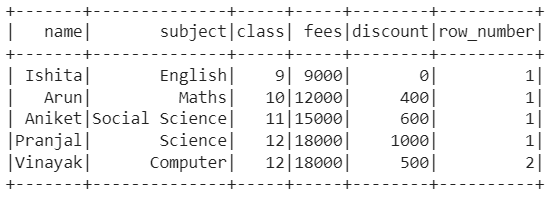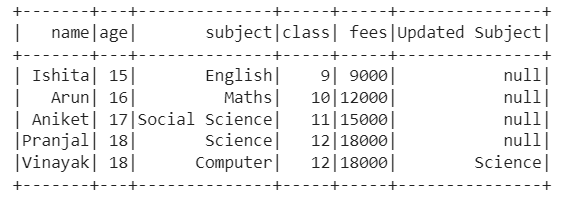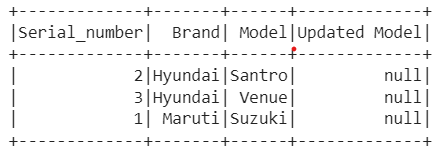Open in App
Not now

# Partitioning by multiple columns in PySpark with columns in a list

• Last Updated : 28 Dec, 2022

Pyspark offers the users numerous functions to perform on the dataset. One such function which seems to be too useful is Pyspark, which operates on group of rows and return single value for every input. Do you know that you can even the partition the dataset through the Window function? Not only partitioning is possible through one column, but you can partition the dataset through various columns. In this article, we will discuss the same, i.e., partitioning by multiple columns in PySpark with columns in a list.

### Modules Required:

Pyspark: An open source, distributed computing framework and set of libraries for real-time, large-scale data processing API primarily developed for Apache Spark, is known as Pyspark.  This module can be installed through the following command in Python:

`pip install pyspark`

## Stepwise Implementation of :

Step 1: First of all, import the required libraries, i.e. SparkSession, and Window. The SparkSession library is used to create the session, while the Window function returns a single value for every input row. Also, you can import any other libraries like functions or row number for the operations you want to perform on the dataset after partitioning by multiple column is done.

```from pyspark.sql import SparkSession
from pyspark.sql.window import Window```

Step 2: Now, create a spark session using the getOrCreate function.

`spark_session = SparkSession.builder.getOrCreate()`

Step 3: Then, read the CSV file and display it to see if it is correctly uploaded.

```data_frame=csv_file = spark_session.read.csv('#Path of CSV file',
sep = ',', inferSchema = True, header = True)```

Step 4: Later on, declare a list of columns according to which partition has to be done.

`column_list = ["#column-1","#column-2"]`

Step 5: Next, partition the data through the columns in the list declared in last step and rearrange the data through any column name using the Window function.

`Windowspec = Window.partitionBy(column_list).orderBy("#column-n")`

Step 6: Finally, perform the action on the partitioned data set whether it is adding row number to the dataset or giving a lag to any column and displaying it in new column.

`data_frame.withColumn("row_number",row_number().over(Windowspec)).show()`

or

```data_frame.withColumn('Updated Column',
func.lag(data_frame['#column-name']).over(Windowspec)).show()```

### Example 1:

In this example, we have used a data frame (link), i.e., a data set of 5×5, on which we applied the window function partition by function through the columns in list declared earlier, i.e., class and fees, and then sort it in ascending order of class. Further, we have added the row number along each entry according to the partitions done and displayed it in new column ‘row_number‘.

## Python3

 `# Python program to partition by multiple``# columns in PySpark with columns in a list`` ` `# Import the SparkSession, Window and row_number libraries``from` `pyspark.sql ``import` `SparkSession``from` `pyspark.sql.window ``import` `Window``from` `pyspark.sql.functions ``import` `row_number`` ` `# Create a spark session using getOrCreate() function``spark_session ``=` `SparkSession.builder.getOrCreate()`` ` `# Read the CSV file``data_frame ``=` `csv_file ``=` `spark_session.read.csv(``    ``'/content/class_data.csv'``, sep``=``','``,``    ``inferSchema``=``True``, header``=``True``)`` ` `# Declare a list according to which partition has to be done``column_list ``=` `[``"class"``, ``"fees"``]`` ` `# Partition data through list elements and sort it``# through class column in Window function``Windowspec ``=` `Window.partitionBy(column_list).orderBy(``"class"``)`` ` `# Create a row number column and assign row numbers``# according to partitionby condition``data_frame.withColumn(``"row_number"``,``                      ``row_number().over(Windowspec)).show()`

Output:### Example 2:

In this example, we have used a data frame (link), i.e., a data set of 5×5, on which we applied the window function partition by function through the columns in list declared earlier, i.e., age, class and fees, and then sort it in ascending order of age. Further, we have added a lag of 1 for each entry of subject and updated it in new column ‘Updated Subject.’

## Python3

 `# Python program to partition by multiple``# columns in PySpark with columns in a list`` ` `# Import the SparkSession, Window and functions libraries``from` `pyspark.sql ``import` `SparkSession``from` `pyspark.sql.window ``import` `Window``import` `pyspark.sql.functions as func`` ` `# Create a spark session using getOrCreate() function``spark_session ``=` `SparkSession.builder.getOrCreate()`` ` `# Read the CSV file``data_frame ``=` `csv_file ``=` `spark_session.read.csv(``    ``'/content/student_data.csv'``, sep``=``','``,``    ``inferSchema``=``True``, header``=``True``)`` ` `# Declare a list according to which partition has to be done``column_list ``=` `[``"age"``, ``"class"``, ``"fees"``]`` ` `# Partition data through list elements and sort it``# through age column in Window function``Windowspec ``=` `Window.partitionBy(column_list).orderBy(``"age"``)`` ` `# Calculating lag of subject by 1 for each student and putting``# in new column 'Updated Subject'``data_frame.withColumn(``'Updated Subject'``, func.lag(``    ``data_frame[``'subject'``]).over(Windowspec)).show()`

Output:### Example 3:

In this example, we have created a data frame using list comprehension with columns ‘Serial Number,’ ‘Brand,’ and ‘Model‘ on which we applied the window function partition by function through the columns in list declared earlier, i.e., Brand, Model, and then sort it in ascending order of Brand. Further, we have added a lag of 1 for each entry of Model and updated it in new column ‘Updated Model.’

## Python3

 `# Python program to partition by multiple``# columns in PySpark with columns in a list`` ` `# Import the SparkSession, Row, Window and functions libraries``from` `pyspark.sql ``import` `SparkSession, Row``from` `pyspark.sql.window ``import` `Window``import` `pyspark.sql.functions as func`` ` `# Create a spark session using getOrCreate() function``spark_session ``=` `SparkSession.builder.getOrCreate()`` ` `# Create the data frame with list comprehension using createDataFrame function``data_frame ``=` `spark_session.createDataFrame([Row(Serial_number``=``1``,``           ``Brand``=``'Maruti'``, Model``=``'Suzuki'``), Row(``           ``Serial_number``=``2``, Brand``=``'Hyundai'``, Model``=``'Santro'``),``           ``Row(Serial_number``=``3``, Brand``=``'Hyundai'``, Model``=``'Venue'``)])`` ` `# Declare a list according to which partition has to be done``column_list ``=` `[``"Brand"``, ``"Model"``]`` ` `# Partition data through list elements and sort it``# through Brand column in Window function``Windowspec ``=` `Window.partitionBy(column_list).orderBy(``"Brand"``)`` ` `# Calculating lag of subject by 1 for each student and``# putting in new column 'Updated Subject'``data_frame.withColumn(``'Updated Model'``, func.lag(``    ``data_frame[``'Model'``]).over(Windowspec)).show()`

Output:My Personal Notes arrow_drop_up